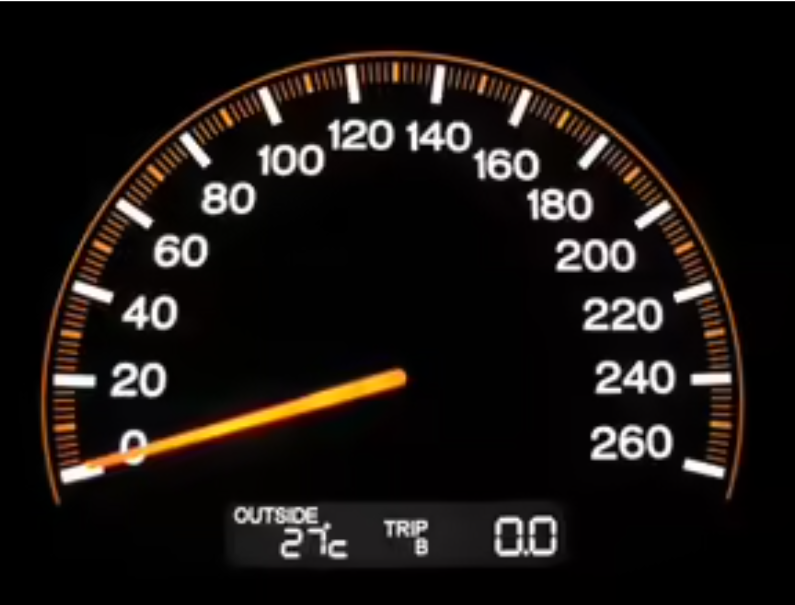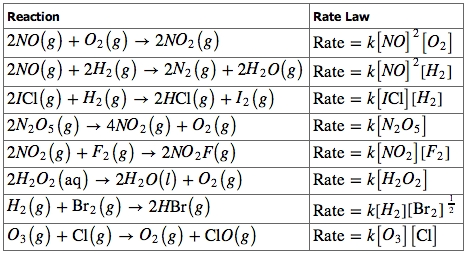# Determining the Rate Law from Experimental Data Study Guide

INTRODUCTION

We have all seen a speedometer. These devices are used to measure the speed of any moving object. We generally see them in our cars or bikes, and they play a very important role by giving us an idea of how fast we are moving and whether we need to slow down (if we are going over the speed limit) or increase our speed if we are in a hurry.

A chemical reaction that’s taking place also has a speed. This tells us how soon a reaction will be completed (all the reactants converted into products). The speed of a chemical reaction is measured by the rate law, which we are going to discuss in detail:Source

### RATE LAW

The mathematical link between the speed of a reaction and the concentrations of the reagents is known as the rate law for a chemical process. For a general equation,

aA+bB → C

With no intermediary stages in its reaction process, the rate is provided by:

r=k[A]x[B]ySource

### ZERO-ORDER REACTIONS

Unlike some of the other orders of reaction, a zero-order reaction has a rate that is independent of the reactant concentration. As a result, raising or reducing the concentration of the reactive species does not affect the rate of the reaction. Zero-order reactions occur when a component that is necessary for the reaction to progress, such as a substrate or a catalyst, is saturated by the reactants.

### FIRST-ORDER REACTIONS

Only one reactant’s concentration determines the outcome of a first-order reaction. As a result, a first-order reaction is often known as a unimolecular reaction.

## CONCLUSION

• The mathematical link between the speed of a reaction and the concentrations of the reagents is known as the rate law for a chemical process.
• A series of experiments with varied initial amounts of reactants must be undertaken to experimentally ascertain a rate law.

### FAQs:

1. How do you determine the rate law?

A rate law depicts how the rate of a chemical reaction is affected by the concentration of reactants. The rate rule for a reaction like aA → product is usually in the form rate = k[A]ⁿ, where k is a proportionality factor termed the rate constant, and n is the reaction order with respect to A.

2. Why is determining a rate law important?

The pace of a chemical reaction is possibly its most crucial attribute since it determines whether a reaction can occur. Using the rate law, which is an equation that connects the rate to the concentrations of reactants, can aid a chemist in fine-tuning the reaction conditions for a better rate.

3. What factors affect rate law?Many factors impact the pace of a chemical reaction, including reactant concentration, surface area, temperature, and catalysts.

We hope you enjoyed studying this lesson and learned something cool about Determining the Rate Law from Experimental Data! Join our Discord community to get any questions you may have answered and to engage with other students just like you! Don’t forget to download our App to experience our fun, VR classrooms – we promise, it makes studying much more fun! 😎

## SOURCES:

1. Determining the Rate Law from Experimental Data. https://www.ck12.org/c/chemistry/determining-the-rate-law-from-experimental-data/lesson/Determining-the-Rate-Law-from-Experimental-Data-CHEM/. Accessed 7 Feb 2022.
2. The Rate Law: Concentration and Time. https://courses.lumenlearning.com/boundless-chemistry/chapter/the-rate-law-concentration-and-time/. Accessed 7 Feb 2022.
]]>